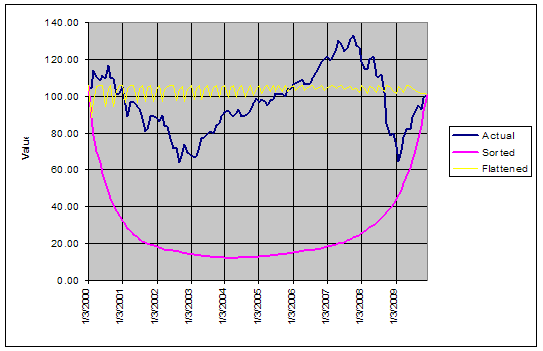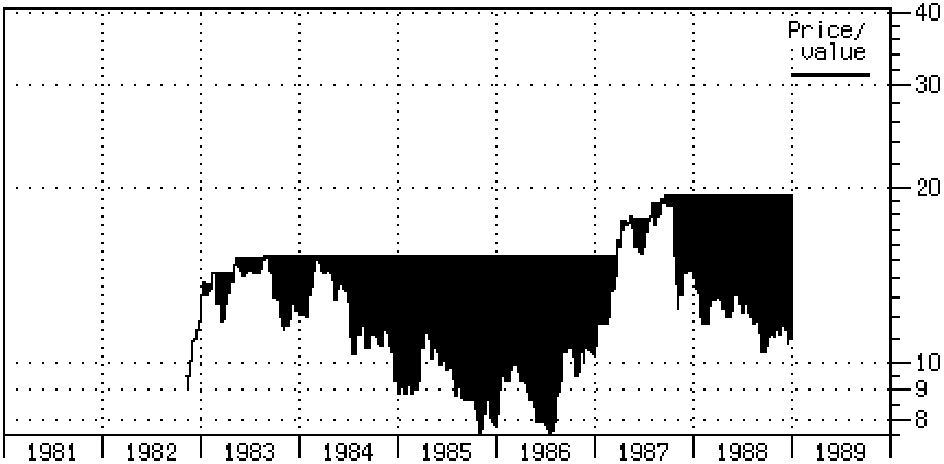## Investment Risk & Risk-Adjusted Performance

by Peter G. Martin

## What is the Ulcer Index?

Ulcer Index (UI) is a method for measuring investment risk that addresses the real concerns of investors, unlike the widely used standard deviation of return (SD). UI is a measure of the depth and duration of drawdowns in prices from earlier highs.

Using UI instead of SD can lead to very different conclusions about investment risk and risk-adjusted return, especially when evaluating strategies that seek to avoid major declines in portfolio value (market timing, dynamic asset allocation, hedge funds, etc.).

The Ulcer Index was originally developed by the author of this page in 1987. Since then, it has been widely recognized and adopted by the investment community. According to Nelson Freeburg, editor of Formula Research, Ulcer Index is “perhaps the most fully realized statistical portrait of risk there is.”

The Index was first described in The Investor's Guide to Fidelity Funds: Winning Strategies for Mutual Fund Investors, by myself and Byron McCann. Originally published by John Wiley & Sons in 1989, this out-of-print book is available in PDF format from http://www.tangotools.com/ui/fkbook.pdf.

Ulcer Index is also explained on Wikipedia at http://en.wikipedia.org/wiki/Ulcer_Index

Note: There have been instances of the term "Ulcer Index" being used for risk measures that do not strictly follow the details described here. This document explains the correct use of the concept.

## What's Wrong with Standard Deviation of Return?

Standard deviation is a statistical measure of the variability or unpredictability of an investment's return. As a measure of risk, it suffers from a number of serious drawbacks:

• Both upward and downward changes in value add to the calculated SD. Real investors associate risk only with the downside. Rising prices create profits, not risk.
• The calculated value of SD is not affected by the sequences in which gains and losses occur. Thus, SD does not recognize the strings of losses that result in significant drawdowns in value. The three hypothetical investments in the chart below have the same annualized return (-0.52%/year) and the same SD (4.66%/month), but no rational investor would consider them as having the same risk.
• ##This chart shows the NAV of an S&P 500 Index mutual fund with dividends reinvested, using monthly data over the 10 years 2000-2009. The Actual (dark blue) line is the fund's actual performance. The Sorted (magenta) line was obtained by re-arranging the monthly percentage returns, so that the worst months occur first and the best ones occur last. The Flattened (yellow) line was obtained by shuffling the monthly returns in such a way as to minimize the depth and duration of drawdowns. The set of monthly returns is the same in each case; only their order has been changed. See http://www.tangotools.com/ui/SD.xls.

• When SD is used to measure the risk of a market timing strategy, it will tell you roughly how often you were out of the market, but nothing about whether you were out at the right times. SD doesn't tell you if your strategy reduced risk by avoiding market downturns.
Because of these weaknesses, SD does not reward an investment strategy for avoiding market downturns. Using Ulcer Index as a risk measure avoids all of these problems.

## What About Other Risk Measures?

Other established risk measures have weaknesses too. For example:
• Some are based on the single worst event over a time period, which by definition has no statistical significance (e.g. Worst Trade and Maximum Drawdown).
• Some are based on absolute rather than percentage price changes, which distorts results over longer periods with strong price trends (e.g. Average Maximum Retracement).
• Some tell you how often you lost money, but not how much (e.g. Percentage Losing Quarters).
• Some cannot be used to compare investment alternatives. For example, Percentage Losing Trades cannot be used to compare a market timing strategy with a buy-and-hold approach, because the latter has no trades.

## How is Ulcer Index Calculated?

Ulcer Index measures the depth and duration of percentage drawdowns in price from earlier highs. The greater a drawdown in value, and the longer it takes to recover to earlier highs, the higher the UI. Technically, it is the square root of the mean of the squared percentage drawdowns in value. The squaring effect penalizes large drawdowns proportionately more than small drawdowns (the SD calculation also uses squaring).

In effect, UI measures the "severity" of drawdowns, as represented by the dark regions in the charts below:Drawdowns in value: S&P 500 index with dividends reinvestedDrawdowns in value: Fidelity Select Precious Metals & Minerals fund

The algorithm for computing UI is simple, and can be seen in the pseudo-code fragment below:

SumSq = 0
MaxValue = 0
for T = 1 to NumOfPeriods do
if Value[T] > MaxValue then MaxValue = Value[T]
else SumSq = SumSq + sqr(100 * ((Value[T] / MaxValue) - 1))
UI = sqrt(SumSq / NumOfPeriods)

An Excel spreadsheet showing how to calculate the Ulcer Index is available at http://www.tangotools.com/ui/UlcerIndex.xls

UI has a further advantage over SD. Its calculated value is essentially the same over a wide range of time intervals between data points. Weekly price data is a robust choice, but daily data can be used as well. As the interval is extended beyond a week, there is an increasing danger of missing significant intra-period drawdown-and-recovery events. The use of quarterly or longer intervals is strongly discouraged for this reason.

Unlike UI, the calculated value of SD depends directly on the time period used. For example, the SD of annual return is roughly 7.2 times the SD of weekly return (7.2 is the square root of 52 weeks per year). Since the time period is often unstated, this creates opportunities for serious misunderstandings about an investment's risk.

## Measuring Investment Performance

A popular method for measuring investment "performance" is to divide the excess return of an investment by its risk. (Excess return is total return minus the return offered by risk-free investments such as money market funds.) This calculation provides a single number that accounts for both return and risk. It reports the additional return achieved per unit of risk assumed. Traditionally the Sharpe Ratio is used, where risk is again represented by the standard deviation of return:
Sharpe Ratio = (Total return - Risk-free return) / SD
Just as SD is a poor risk measure, so is this formula a poor performance measure. This problem is solved by simply replacing SD with UI. This new performance measure is known as the "Ulcer Performance Index" (UPI) or "Martin Ratio".
UPI = (Total return - Risk-free return) / UI

When plotting investments on a risk vs return chart, UI can be used instead of SD for the horizontal (risk) axis.If a line is drawn between the points representing the risk-free return and a risky investment, the slope of the line is equal to the Ulcer Performance Index. As with the Sharpe Ratio, if an investment lies above the line joining the risk-free return with the S&P 500, the investment is "beating the market" on a risk-adjusted basis.

## Market Timing Example

The table below shows the results achieved with both UI and SD. We compared two strategies over the period 1940-1997: buy-and-hold the S&P 500 index, and timing the index with a simple momentum indicator. Results include reinvestment of dividends in both cases.

 Buy-and-Hold Timing System % Change Annualized Total Return (%/yr) 12.59 14.79 17.5 Ulcer Index (%) 8.85 5.14 -41.9 Ulcer Performance Index (UPI) 0.92 2.01 118.9 Standard Deviation (%/yr) 16.10 13.18 -18.1 SD Performance (Sharpe Ratio) 0.51 0.78 52.9

For the timing system, annualized total return is increased by a modest 2.2 percentage points over buy-and-hold. SD reports 18% lower risk and 53% higher performance (Sharpe Ratio). UI reports 42% lower risk and 119% higher performance (UPI). Thus, UI places a much higher value on the market timing system than does SD.

Other experimental work has shown that many popular market timing systems have little value when SD is used to measure risk, but significant value when UI is used instead. This arises because SD fails to recognize the success of timing systems in avoiding major market downturns.

## Caveat Emptor

With any method for computing investment risk and performance, it is important to use data covering as long a time period as possible. In particular, the measurement period must include both bull and bear markets for the investments of interest. Needless to say, when comparing multiple investments, the same time period must be used in each case.

All risk and return calculations should include reinvestment of interest, dividends and other distributions; and should be net of all recurring fees, transaction costs and trading slippage.

Any use of Ulcer Index or UPI that falls outside of these constraints would be inappropriate and of little value.